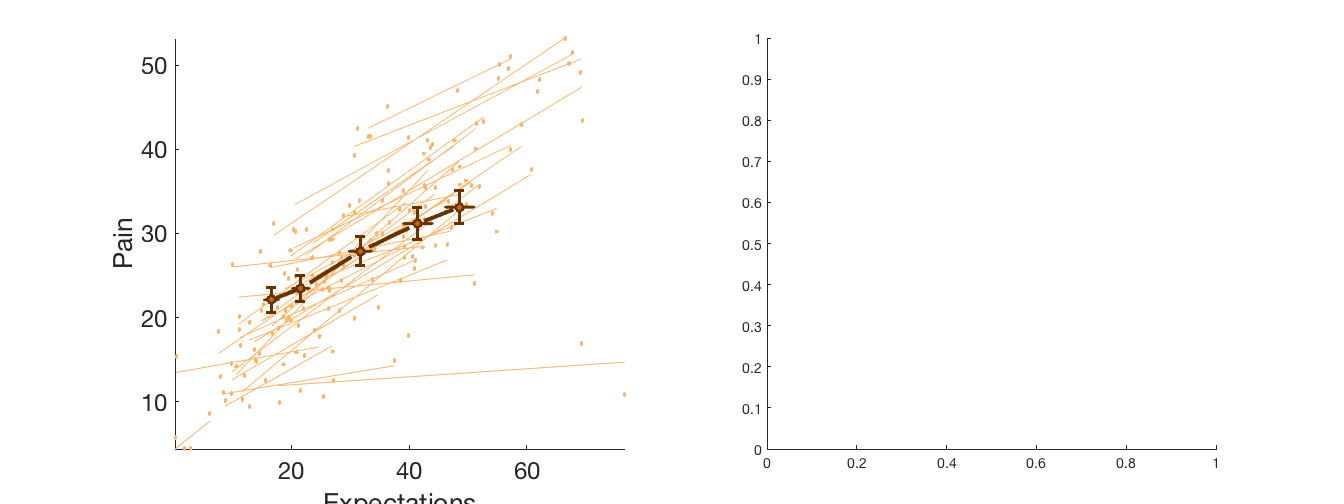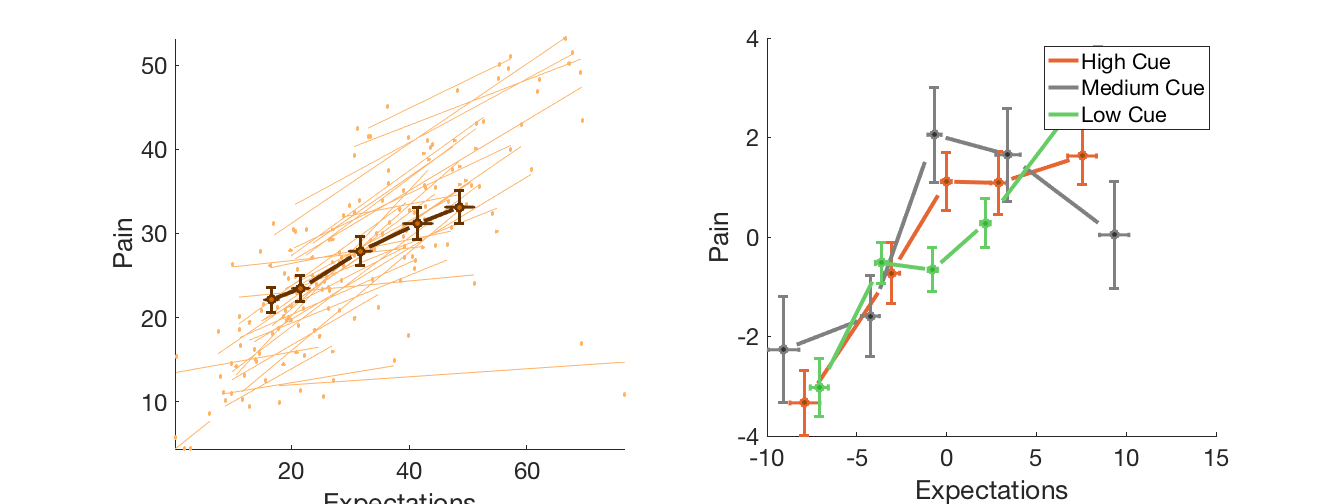## Dependencies:

```% Matlab statistics toolbox
% Matlab signal processing toolbox
% Statistical Parametric Mapping (SPM) software https://www.fil.ion.ucl.ac.uk/spm/
% For full functionality, the full suite of CANlab toolboxes is recommended. See here: Installing Tools```

## Canlab dataset basic usage

Shows how to load a sample canlab_dataset object, extract variables, and make some plots.

```% Instructions to include in help files as appropriate:
% -----------------------------------------------------------------------
%
% Before you start, the CANlab_Core_Tools must be added to your path with
% subfolders. Otherwise, you will get errors.
%
% Sample datasets are in the "Sample_datasets" folder in CANlab_Core_Tools.
%
% This example will use behavioral data in the file:
% 'Jepma_IE2_single_trial_canlab_dataset.mat'
% The dataset is a multi-level dataset with 34 subjects, 2 subject-level vars, 10 event-level vars
% consisting of a time series of trial-by-trial ratings of pain, expectations, and cue values for each subject
%
% These data have been submitted for publication (M. Jepma, Wager et al.)
% Please do not reuse without permission.
```

## Load the sample dataset and print a summary

```% Load sample data file (this is in Sample_datasets in Core - make sure it's on your path)

% Print a summary:
```
```
--------- DATASET VARS --------

Experiment name:
IE2 : Marieke Jepma

34 subjects, 2 subject-level vars, 10 event-level vars
Warning: Missing variable type for variable MeanPain. Type assumed to be
numeric.
Warning: Missing variable type for variable MeanNPS. Type assumed to be numeric.

--------- SUBJECT LEVEL VARS: data_obj.Subj_Level --------

Var_name     Description
__________    ___________

'MeanPain'    'MeanPain'
'MeanNPS'     'MeanNPS'

Warning: Missing variable type for variable trial. Type assumed to be numeric.
Warning: Missing variable type for variable temp. Type assumed to be numeric.
Warning: Missing variable type for variable cue. Type assumed to be numeric.
Warning: Missing variable type for variable cue valence. Type assumed to be
numeric.
Warning: Missing variable type for variable expected pain. Type assumed to be
numeric.
Warning: Missing variable type for variable reported pain. Type assumed to be
numeric.
Warning: Missing variable type for variable vif. Type assumed to be numeric.
Warning: Missing variable type for variable NPS. Type assumed to be numeric.
Warning: Missing variable type for variable Expected pain next trial. Type
assumed to be numeric.
Warning: Missing variable type for variable Pain next trial. Type assumed to be
numeric.

--------- EVENT LEVEL VARS: data_obj.Event_Level --------

Var_name                                    Description
__________________________    __________________________________________________________

'trial'                       'Trial number'
'temp'                        'Temperature: -0.5 = 48 degrees, 0.5 = 49 degrees'
'cue'                         'Cue identity: 1-3 = CSlow, Cue 4-6 = CShigh, 7 = neutral'
'cue valence'                 'Cue valence: -.5 low +.5 high'
'expected pain'               'Expected pain'
'reported pain'               'Reported pain'
'vif'                         'Variance inflation factor for trial'
'NPS'                         'NPS response'
'Expected pain next trial'    'Expected pain on next trial'
'Pain next trial'             'Reported pain on next trial'

Warning: Missing variable type for variable MeanPain. Type assumed to be
numeric.
Warning: Missing variable type for variable MeanNPS. Type assumed to be numeric.
Warning: Missing variable type for variable trial. Type assumed to be numeric.
Warning: Missing variable type for variable temp. Type assumed to be numeric.
Warning: Missing variable type for variable cue. Type assumed to be numeric.
Warning: Missing variable type for variable cue valence. Type assumed to be
numeric.
Warning: Missing variable type for variable expected pain. Type assumed to be
numeric.
Warning: Missing variable type for variable reported pain. Type assumed to be
numeric.
Warning: Missing variable type for variable vif. Type assumed to be numeric.
Warning: Missing variable type for variable NPS. Type assumed to be numeric.
Warning: Missing variable type for variable Expected pain next trial. Type
assumed to be numeric.
Warning: Missing variable type for variable Pain next trial. Type assumed to be
numeric.

--------- SUBJECT LEVEL VARS: data_obj.Subj_Level --------

Var_name     Min_value    Max_value    Mean_value    St_Dev     IQR      NaN_count
__________    _________    _________    __________    ______    ______    _________

'MeanPain'      5.8015      46.911         27.45      9.5331    12.429        0
'MeanNPS'     -0.72369      87.081        32.079      20.682    27.863        0

--------- EVENT LEVEL VARS: data_obj.Event_Level --------

Var_name             Min_value    Max_value    Mean_value    St_Dev       IQR      NaN_count
__________________________    _________    _________    __________    _______    _______    _________

'trial'                              1           70           35.4     20.231         35       48
'temp'                        -0.54286      0.52857     -0.0025484    0.49995          1       48
'cue'                                1            7         4.0039     2.0048          4       48
'cue valence'                       -1            1              0    0.92536          2       48
'expected pain'                      0       81.333         31.975      16.78     23.533       48
'reported pain'                      0         67.8         27.503     13.149     18.333       48
'vif'                           1.0589       2.9802         1.3221     0.2779    0.19197       48
'NPS'                          -187.75       273.47         32.208     44.535     52.665       78
'Expected pain next trial'           0       81.333         31.976     16.755     23.533       82
'Pain next trial'                    0         67.8         27.549     13.166     18.467       82

```

## Extract data two variables and make a plot

```% Extract expectation and pain ratings, two continuous event-level variables from the object
[~, expect] = get_var(DAT, 'expected pain');
[~, pain] = get_var(DAT, 'reported pain');

% Make a multi-level scatterplot
create_figure('lines_expect_pain', 1, 2);
[han, Xbin, Ybin] = line_plot_multisubject(expect, pain, 'n_bins', 5, 'group_avg_ref_line', 'MarkerTypes', 'o', 'colors', custom_colors([1 .7 .4], [1 .7 .4], 100), 'gcolors', {[.4 .2 0] [.8 .4 0]});
axis tight
xlabel('Expectations'); ylabel('Pain'); set(gca, 'FontSize', 24);
```
```Warning: Missing variable type for variable expected pain. Type assumed to be
numeric.
Warning: Missing variable type for variable reported pain. Type assumed to be
numeric.
r = 0.76, t( 33) = 12.53, p = 0.000000, num. missing:   2
```## Extract data from one variable conditional on another variable, and plot

```% Now extract expectation and pain variables conditional on cue value:
[~, expect_cshigh] = get_var(DAT, 'expected pain', 'conditional', {'cue valence' 1});
[~, expect_csmed] = get_var(DAT, 'expected pain', 'conditional', {'cue valence' 0});
[~, expect_cslow] = get_var(DAT, 'expected pain', 'conditional', {'cue valence' -1});
[~, pain_cshigh] = get_var(DAT, 'reported pain', 'conditional', {'cue valence' 1});
[~, pain_csmed] = get_var(DAT, 'reported pain', 'conditional', {'cue valence' 0});
[~, pain_cslow] = get_var(DAT, 'reported pain', 'conditional', {'cue valence' -1});

% Now make the plot, without individual subject lines
% First set colors and condition names
color1 = {[.9 .4 .2] [.6 .3 0]};
color2 = {[.5 .5 .5] [.2 .2 .2]};
color3 = {[.4 .8 .4] [.2 .7 .2]};
condition_names = {'High Cue' 'Medium Cue' 'Low Cue'};

% Now plot:
subplot(1, 2, 2)
[han1, Xbin, Ybin] = line_plot_multisubject(expect_cshigh, pain_cshigh, 'n_bins', 5, 'group_avg_ref_line', 'MarkerTypes', 'o', 'colors', color1, 'center', 'noind', 'nolines');
[han2, Xbin, Ybin] = line_plot_multisubject(expect_csmed, pain_csmed, 'n_bins', 5, 'group_avg_ref_line', 'MarkerTypes', 'o', 'colors',color2, 'center', 'noind', 'nolines');
[han3, Xbin, Ybin] = line_plot_multisubject(expect_cslow, pain_cslow, 'n_bins', 5, 'group_avg_ref_line', 'MarkerTypes', 'o', 'colors',color3, 'center', 'noind', 'nolines');
xlabel('Expectations'); ylabel('Pain'); set(gca, 'FontSize', 24);
legend([han1.grpline_handle(1) han2.grpline_handle(1) han3.grpline_handle(1)], condition_names);
drawnow, snapnow
```
```Warning: Missing variable type for variable expected pain. Type assumed to be
numeric.
Warning: Missing variable type for variable expected pain. Type assumed to be
numeric.
Warning: Missing variable type for variable expected pain. Type assumed to be
numeric.
Warning: Missing variable type for variable reported pain. Type assumed to be
numeric.
Warning: Missing variable type for variable reported pain. Type assumed to be
numeric.
Warning: Missing variable type for variable reported pain. Type assumed to be
numeric.
r = 0.54, t( 33) = 3.64, p = 0.000919, num. missing:   3
r = 0.24, t( 33) = 1.43, p = 0.161345, num. missing:   3
r = 0.59, t( 33) = 5.64, p = 0.000003, num. missing:   2
```